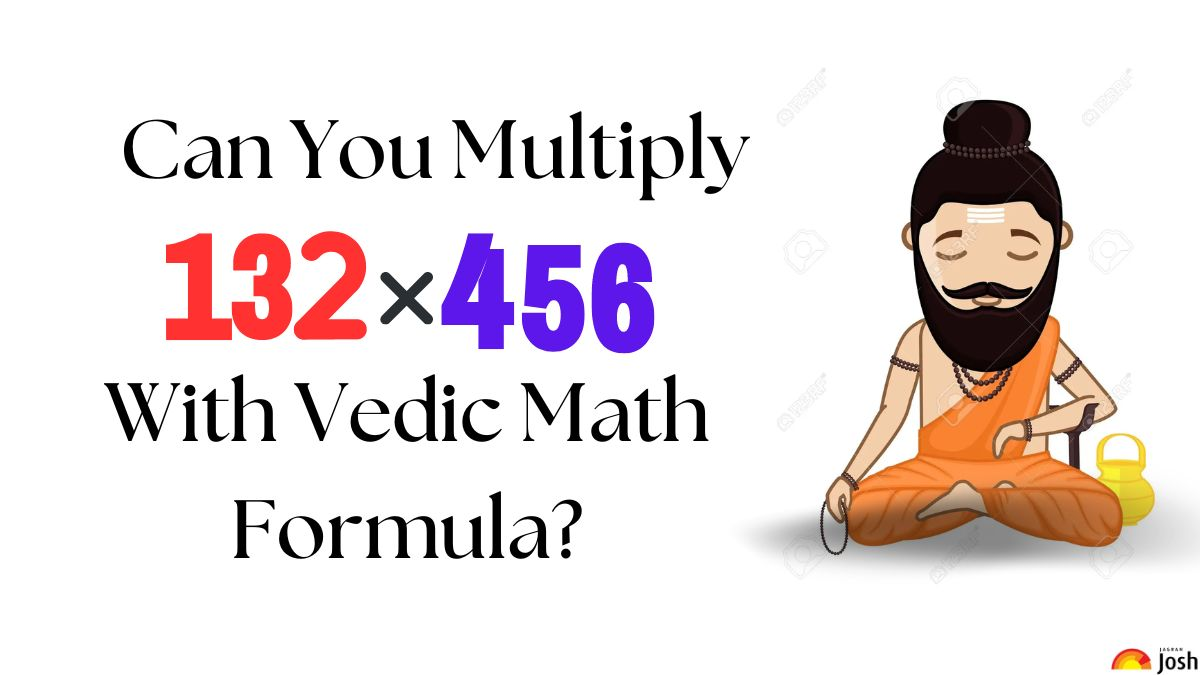# Vedic Math Puzzle: Only 1% Of Genius Can Find The Product of The Two Digits Below With Vedic Sutra in 19 Seconds. Hurry Up!

Vedic Math Puzzle: Vedic math involves learning mental calculation methods, shortcuts, and patterns to perform arithmetic operations, solve complex equations, and make calculations easier. Try this one!Do you know the formula for this Vedic Equation?

Vedic Math Puzzle: Vedic math puzzles are mathematical problems or equations that use methods from the ancient Indian system of Vedic mathematics to solve them more quickly and effectively.

Vedic math puzzles are vital because they enhance general mathematical ability and cultivate a respect for Vedic mathematics while encouraging critical thinking, problem-solving abilities, and mental agility. Your task in this equation is to use a Vedic Math formula to calculate the product of the two units.

Look for the equation below:

Do You Think You Will Be Able To Solve?

Don’t Say It’s Not Your Cup Of Tea!

What is Vedic Math?

Vedic math multiplication is a collection of methods that were derived from classical Indian mathematics that provide quick and different ways to multiply integers. These techniques help to speed up and simplify calculations while simplifying challenging multiplication problems.

Are You Skilled Enough To Solve Basic Multiplication Using Vedic Formula in 27 Seconds?

Hey Genius…

You are Losing…

The benefits of solving Vedic math equations are numerous. Math becomes more interesting and approachable as a result of these improvements in mental calculation abilities, speed and precision of mathematical operations, logical thinking and problem-solving skills, and alternate approaches to hard computations.

Tick…

Tock…

Tick…

I am sure you have a digit. Just tally your steps and answers with the below picture.

Understand the steps here:

Step 1: 6*2 = 12 (write 2, carry 1)

Step 2: 6*3 + 2*5 = 28 + 1 = 29 (write 9, carry 2)

Step 3: 6*1 + 3*5 + 2*4 + 2 (carried) = 31 (write 1, carry 3)

Step 4: 1*5 + 3*4 = 17 + 3 (carried) = 20 (write 0, carry 2)

Step 5: 1*4 = 4 + 2 = 6 (write 6)

Therefore, the correct answer to this is 60192.

This three-digit multiplication equation is solved using the Sutra (formula) of Urdhva-Tiryagbyham. It is a Vedic math technique that involves vertical and crosswise multiplication, enabling efficient multiplication of multi-digit numbers by breaking them down into simpler computations.

You did a great job. Scroll back to JagranJosh to discover more about Vedic Maths and its various Sutras.

Only A Record-Holding Genius Can Find The Odd Digit in 3 Seconds. Try Your Skills!

Get the latest General Knowledge and Current Affairs from all over India and world for all competitive exams.
खेलें हर किस्म के रोमांच से भरपूर गेम्स सिर्फ़ जागरण प्ले पर